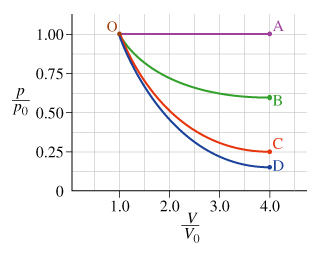# Problem: An ideal monatomic gas is contained in a cylinder with a movable piston so that the gas can do work on the outside world, and heat can be added or removed as necessary. The figure shows various paths that the gas might take in expanding from an initial state whose pressure, volume, and temperature are p0, V0, and T0 respectively. The gas expands to a state with final volume 4V0. For some answers it will be convenient to generalize your results by using the variable Rv = Vfinal/Vinitial, which is the ratio of final to initial volumes (equal to 4 for the expansions shown in the figure.)The figure shows several possible paths of the system in the pV plane. Although there are an infinite number of paths possible, several of those shown are special because one of their state variables remains constant during the expansion. These have the following names:Adiabiatic: No heat is added or removed during the expansion.Isobaric: The pressure remains constant during the expansion.Isothermal: The temperature remains constant during the expansion.Part DGraphically, the work along any path in the pV plot ____________.a) is the area to the left of the curve from P0 to Pfinal.b) is the area under the curve from V0 to Vfinalc) requires knowledge of the temperature T(V)

###### FREE Expert Solution

Part D

In a thermodynamic process, the work is done is:

$\overline{){\mathbf{W}}{\mathbf{=}}{{\mathbf{\int }}}_{{\mathbf{V}}_{\mathbf{i}}}^{{\mathbf{V}}_{\mathbf{f}}}{\mathbf{p}}{\mathbf{d}}{\mathbf{V}}}$

83% (382 ratings)###### Problem Details

An ideal monatomic gas is contained in a cylinder with a movable piston so that the gas can do work on the outside world, and heat can be added or removed as necessary. The figure shows various paths that the gas might take in expanding from an initial state whose pressure, volume, and temperature are p0, V0, and T0 respectively. The gas expands to a state with final volume 4V0. For some answers it will be convenient to generalize your results by using the variable Rv = Vfinal/Vinitial, which is the ratio of final to initial volumes (equal to 4 for the expansions shown in the figure.)

The figure shows several possible paths of the system in the pV plane. Although there are an infinite number of paths possible, several of those shown are special because one of their state variables remains constant during the expansion. These have the following names:

• Isobaric: The pressure remains constant during the expansion.
• Isothermal: The temperature remains constant during the expansion.Part D

Graphically, the work along any path in the pV plot ____________.

a) is the area to the left of the curve from P0 to Pfinal.

b) is the area under the curve from V0 to Vfinal

c) requires knowledge of the temperature T(V)